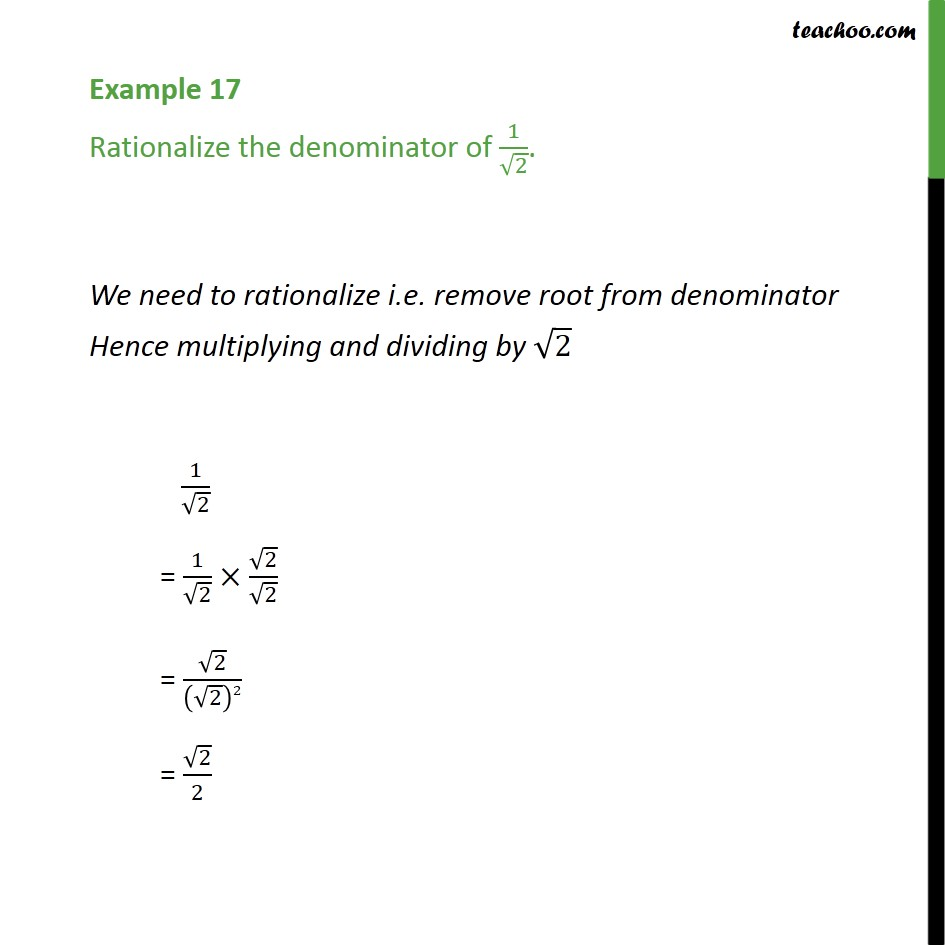1. Chapter 1 Class 9 Number Systems (Term 1)
2. Serial order wise
3. Examples

Transcript

Example 17 Rationalize the denominator of 1/√2. We need to rationalize i.e. remove root from denominator Hence multiplying and dividing by √2 1/√2 = 1/√2 ×√2/√2 = √2/(√2)2 = √2/2